Static Equilibrium for a Particle

A particle: An object with inertia (mass) but of negligible dimensions

Equilibrium equations for a particle: A particle is in equilibrium if the resultant of ALL forces acting on the particle is equal to zero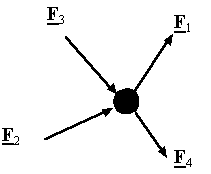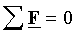Equilibrium equations in component form: In a rectangular coordinate system the equilibrium equations can be represented by three scalar equations: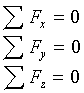Free-Body diagram: A diagram showing the particle under consideration and all the forces acting on this particle. Each force in this diagram must labeled.

String or cable: A mechanical device that can only transmit a tensile force along itself.Linear spring: A mechanical device which exerts a force along its line of action and proportional to its extension.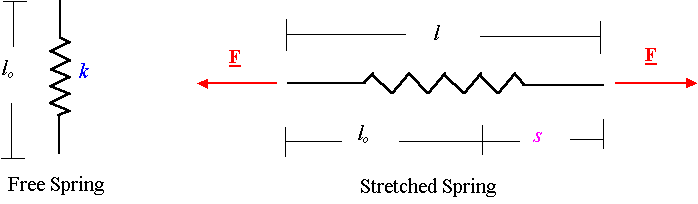Frictionless pulleys: For a frictionless pulley in static equilibrium, the tension in the cable is the same on both sides of the pulley.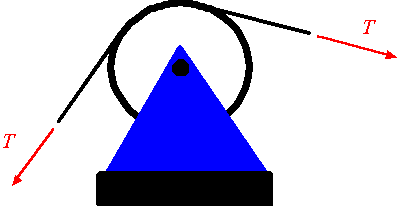Examples: